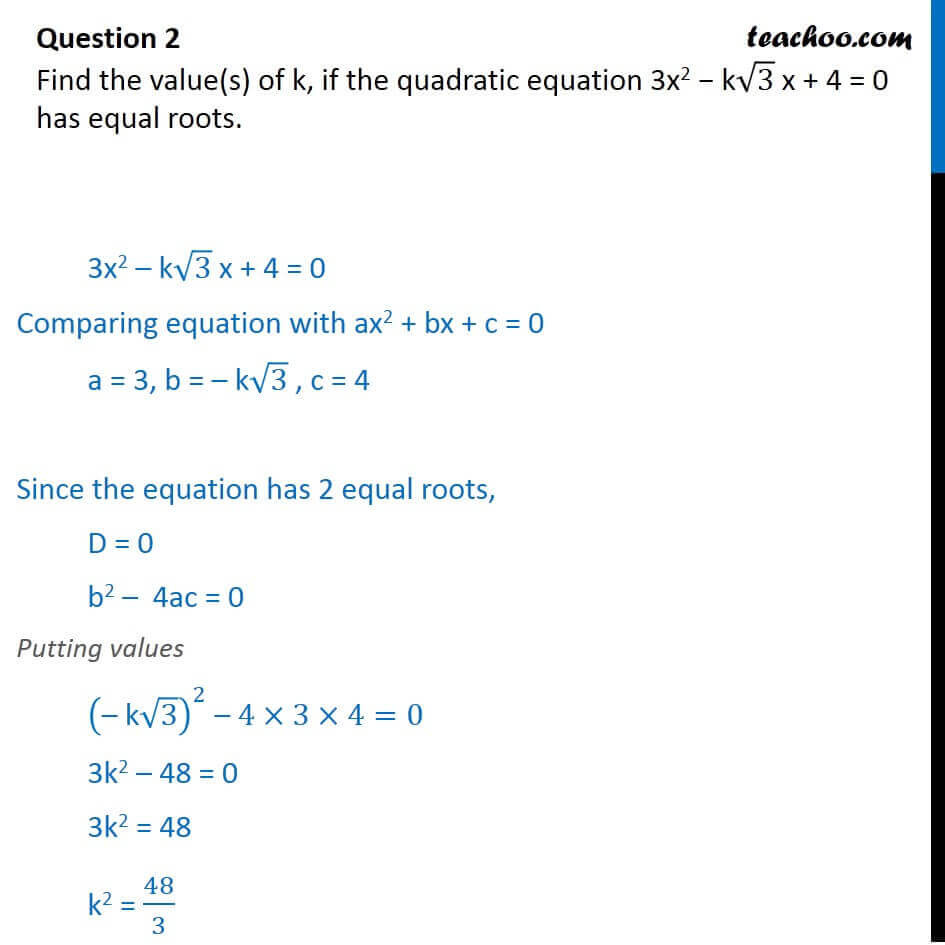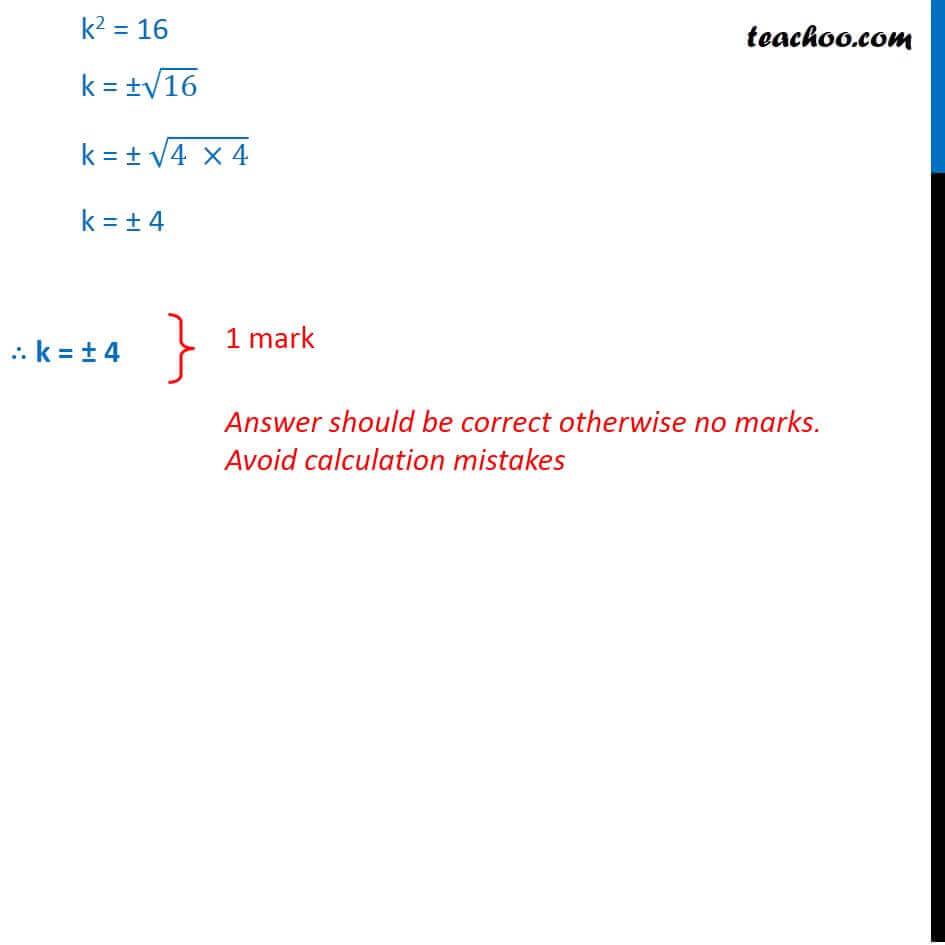CBSE Class 10 Sample Paper for 2018 Boards

Class 10
Solutions of Sample Papers for Class 10 Boards

### Find the value(s) of k, if the quadratic equation 3x 2 − k√3 x + 4 = 0 has equal roots.

This is a question of CBSE Sample Paper - Class 10 - 2017/18.Learn in your speed, with individual attention - Teachoo Maths 1-on-1 Class

### Transcript

Question 2 Find the value(s) of k, if the quadratic equation 3x2 k 3 x + 4 = 0 has equal roots. 3x2 k 3 x + 4 = 0 Comparing equation with ax2 + bx + c = 0 a = 3, b = k 3 , c = 4 Since the equation has 2 equal roots, D = 0 b2 4ac = 0 Putting values (" k" 3)^2 4 3 4=0 3k2 48 = 0 3k2 = 48 k2 = 48/3 k2 = 16 k = 16 k = (4 4) k = 4 k = 4